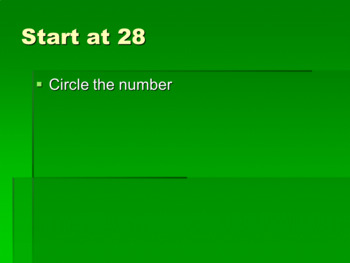# CGI What's My Number? Grid Hunt 2-Lesson BundleSubject
Resource Type
File Type

Zip

(103 KB)
Product Rating
Standards
• Product Description
• StandardsNEW

Use CGI strategies while your students 1) Follow clues on 2 different slide shows to find the missing number on a 100 grid and 2) Solve a CGI word problem. Common Core math vocabulary is featured, as well as opportunities for students to share their answers and find any errors they make. This bundle includes 2 days worth of practice with addition and subtraction skills (numbers to 40 and numbers to 120). Great formative assessment opportunity!

Determine the unknown whole number in an addition or subtraction equation relating three whole numbers. For example, determine the unknown number that makes the equation true in each of the equations 8 + ? = 11, 5 = ▯ - 3, 6 + 6 = ▯.
Subtract multiples of 10 in the range 10-90 from multiples of 10 in the range 10-90 (positive or zero differences), using concrete models or drawings and strategies based on place value, properties of operations, and/or the relationship between addition and subtraction; relate the strategy to a written method and explain the reasoning used.
Given a two-digit number, mentally find 10 more or 10 less than the number, without having to count; explain the reasoning used.
Add within 100, including adding a two-digit number and a one-digit number, and adding a two-digit number and a multiple of 10, using concrete models or drawings and strategies based on place value, properties of operations, and/or the relationship between addition and subtraction; relate the strategy to a written method and explain the reasoning used. Understand that in adding two-digit numbers, one adds tens and tens, ones and ones; and sometimes it is necessary to compose a ten.
The numbers from 11 to 19 are composed of a ten and one, two, three, four, five, six, seven, eight, or nine ones.
Total Pages
N/A
N/A
Teaching Duration
45 minutes
Report this Resource to TpT
Reported resources will be reviewed by our team. Report this resource to let us know if this resource violates TpT’s content guidelines.